## Monday, February 22, 2010

### Coin flips and names (Evil problems in probability continued)

In my post about the girl-named-Florida problem, there is a factor in the analysis looking at the probability of having a girl named Florida given that you have two girls: P(F|2g).

This term is easily calculated as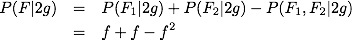which I used in the analysis.

Someone raised the question, "What would happen if (as we know) people don't tend to name two children the same (unless you're George Foreman)?" At first, this seems exactly like a coin flip problem: what is the probability of, in two coin flips, flipping heads on the first flip or flipping heads on the second but not both? It turns out that this is a different problem, and the result is surprising (at least to me). We have to be very careful what information we condition on, knowing that the English language is a little more fluid than we like when dealing with such problems. In the coin flip case we define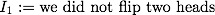:

and it follows, given the probability of flipping heads is h,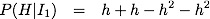which is just the standard result, subtracting off the possibility of having both heads. For h=0.5, this yields the standard result of P(h) = 0.5. As h gets close to 1, the probability of a heads goes way up, and thus the probability of both being heads goes way up. As a result, the probability of just having 1 heads goes to zero.

The situation with names is nearly the opposite: as the frequency of a name increases, the name is much more common. This makes it more and more likely that you will have someone with that name. The difference is in the conditioning information: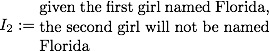The analysis then goes: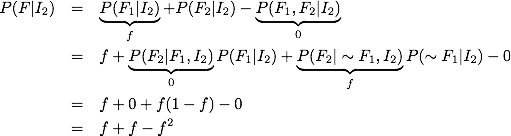which is exactly the same result as the case where one can name both of the children Florida! I was a little surprised by this result, but a quick simulation confirmed it as well.

### Simulation

```from pylab import *
from numpy import *

f=0.1
N=10000
r1=rand(N)
r2=rand(N)

N1=list(r1< f)
N2=list(r2< f)

case1=[n1 or n2 for n1,n2 in zip(N1,N2)]

print "Fraction allowing duplicate names: ",case1.count(True)/float(len(case1))
print "Theoretical Value: ",f+f-f**2

nn=[]
for n1,n2 in zip(N1,N2):
if n1:
nn.append(False)
else:
nn.append(n2)

N2=nn
case2=[n1 or n2 for n1,n2 in zip(N1,N2)]

print "Fraction not allowing duplicate names: ",case2.count(True)/float(len(case2))

```

### Simulation Result

Fraction allowing duplicate names: 0.1853
Theoretical Value: 0.19
Fraction not allowing duplicate names: 0.1853

1.I respectfully disagree with your analysis. For clarity, consider the
2 coins where p(H) = h. Given I_1 that you did not produce 2 heads,
the various combinations are HT, TH, and TT. But, P(HT) = P(TH) =
h*(1-h), and P(TT) = (1-h)^2. Then P(H|I_1) is

P(HT) + P(TH)
-----------------------
P(HT) + P(TH) + P(TT)

or

2h(1-h)
-------------------
2h(1-h) + (1-h)^2

or

2h
----------
1 + h

This has the expected values at h=0, 1/2, and 1. You formula

2h - 2h^2

does not have the correct value near h=1. Remember, you are given the
pre-condition that the population consists of 2 coin pairs where at
least one of the coins is a tail. For h close to 1, elements of
such a population containing 2 tails is extremely rare with almost all
of the pairs consisting of one H and one T. Hence, the probability
must of having a heads in such a population must approach 1, as h
2.